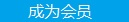您可以捐助，支持我们的公益事业。 1元 10元 50元 认证码：必填求知 文章 文库 Lib 视频 Code iProcess 课程 角色 咨询 工具 火云堂 讲座吧 建模者要资料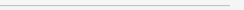订阅捐助
BP神经网络与Python实现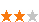6297 次浏览     评价： 好 中 差
2018-3-5

 编辑推荐: 本文来自于cnblogs,人工神经网络是一种经典的机器学习模型，随着深度学习的发展神经网络模型日益完善.

BP神经网络原理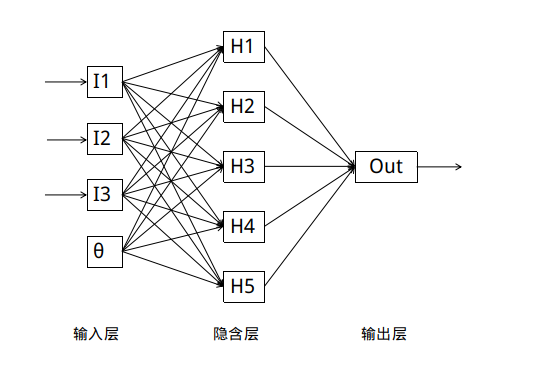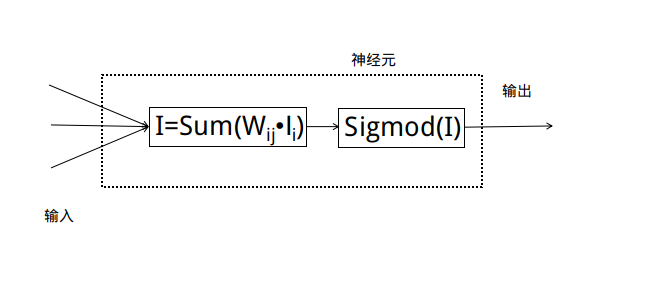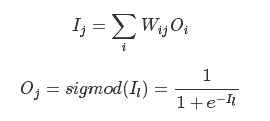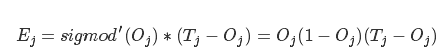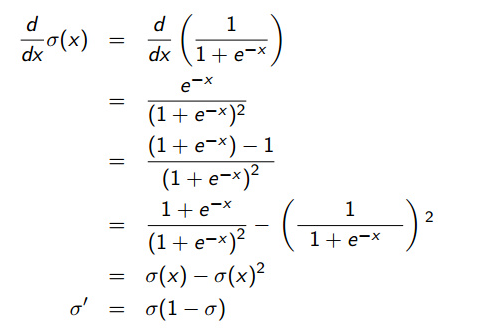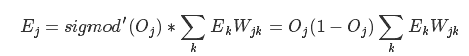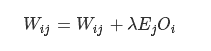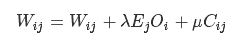μμ是一个称为矫正率的参数.随后更新矫正矩阵: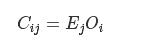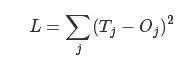Python实现BP神经网络

 def rand(a， b): return (b - a) * random.random() + a def make_matrix(m， n， fill=0.0): # 创造一个指定大小的矩阵 mat = [] for i in range(m): mat.append([fill] * n) return mat

 def sigmoid(x): return 1.0 / (1.0 + math.exp(-x)) def sigmod_derivate(x): return x * (1 - x)

 def setup(self， ni， nh， no): self.input_n = ni + 1 self.hidden_n = nh self.output_n = no # init cells self.input_cells = [1.0] * self.input_n self.hidden_cells = [1.0] * self.hidden_n self.output_cells = [1.0] * self.output_n # init weights self.input_weights = make_matrix(self.input_n， self.hidden_n) self.output_weights = make_matrix(self.hidden_n， self.output_n) # random activate for i in range(self.input_n): for h in range(self.hidden_n): self.input_weights[i][h] = rand(-0.2， 0.2) for h in range(self.hidden_n): for o in range(self.output_n): self.output_weights[h][o] = rand(-2.0， 2.0) # init correction matrix self.input_correction = make_matrix(self.input_n， self.hidden_n) self.output_correction = make_matrix(self.hidden_n， self.output_n)

 def predict(self， inputs): # activate input layer for i in range(self.input_n - 1): self.input_cells[i] = inputs[i] # activate hidden layer for j in range(self.hidden_n): total = 0.0 for i in range(self.input_n): total += self.input_cells[i] * self.input_weights[i][j] self.hidden_cells[j] = sigmoid(total) # activate output layer for k in range(self.output_n): total = 0.0 for j in range(self.hidden_n): total += self.hidden_cells[j] * self.output_weights[j][k] self.output_cells[k] = sigmoid(total) return self.output_cells[:]

 def back_propagate(self， case， label， learn， correct): # feed forward self.predict(case) # get output layer error output_deltas = [0.0] * self.output_n for o in range(self.output_n): error = label[o] - self.output_cells[o] output_deltas[o] = sigmod_derivate(self.output_cells[o]) * error # get hidden layer error hidden_deltas = [0.0] * self.hidden_n for h in range(self.hidden_n): error = 0.0 for o in range(self.output_n): error += output_deltas[o] * self.output_weights[h][o] hidden_deltas[h] = sigmod_derivate(self.hidden_cells[h]) * error # update output weights for h in range(self.hidden_n): for o in range(self.output_n): change = output_deltas[o] * self.hidden_cells[h] self.output_weights[h][o] += learn * change + correct * self.output_correction[h][o] self.output_correction[h][o] = change # update input weights for i in range(self.input_n): for h in range(self.hidden_n): change = hidden_deltas[h] * self.input_cells[i] self.input_weights[i][h] += learn * change + correct * self.input_correction[i][h] self.input_correction[i][h] = change # get global error error = 0.0 for o in range(len(label)): error += 0.5 * (label[o] - self.output_cells[o]) ** 2 return error

 def train(self， cases， labels， limit=10000， learn=0.05， correct=0.1): for i in range(limit): error = 0.0 for i in range(len(cases)): label = labels[i] case = cases[i] error += self.back_propagate(case， label， learn， correct)

 def test(self): cases = [ [0， 0]， [0， 1]， [1， 0]， [1， 1]， ] labels = [， ， ， ] self.setup(2， 5， 1) self.train(cases， labels， 10000， 0.05， 0.1) for case in cases: print(self.predict(case))6297 次浏览  评价: 好 中 差订阅捐助
 相关文章 我们该如何设计数据库 数据库设计经验谈 数据库设计过程 数据库编程总结
 相关文档 数据库性能调优技巧 数据库性能调整 数据库性能优化讲座 数据库系统性能调优系列每天2个文档/视频 扫描微信二维码订阅
 订阅技术月刊 获得每月300个技术资源